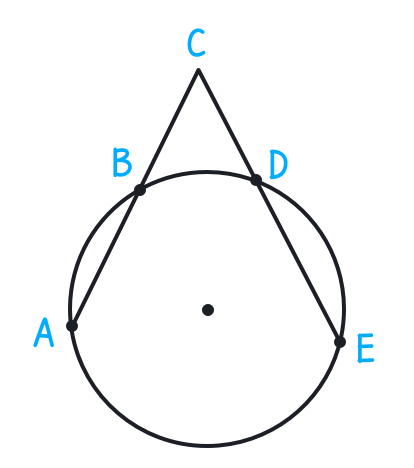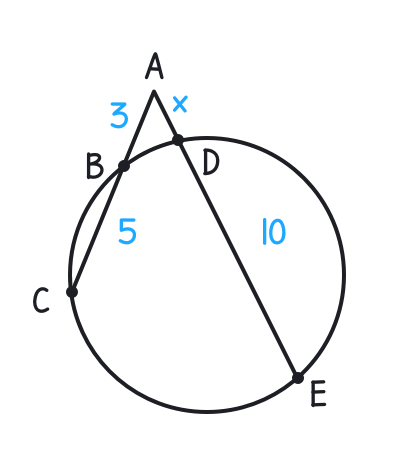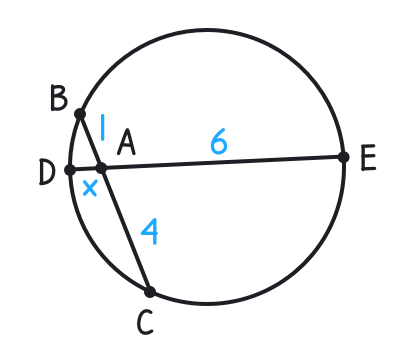### Still have math questions?

Scientific Notation Length Conversion Area Conversion

### Calculator

The Chord Theorem
The Tangent-Secant Theorem
The Intersecting Secants TheoremAE · CE = DE · BE
AE
CE
DE
BE

#### Knowledge

In elementary plane geometry, the power of a point is a real number h that reflects the relative distance of a given point from a given circle.AE · CE = DE · BEBA2 = BC · BDCB · CA = CD · CE

#### Example 1

Find the value of x in the following diagram:AB · AC = AD · AE

3 · (3+5) = x(x+10)

x2 + 10x - 24 = 0

(x+12)(x-2) = 0

x = 2

#### Example 2

Find the value of x in the following diagram:EA · DA = CA · BA

6 · x = 4 · 1

x = 23

Please provide numbers only. Please provide at least two numbers. Please provide 2 to 10 non-zero integer numbers. None